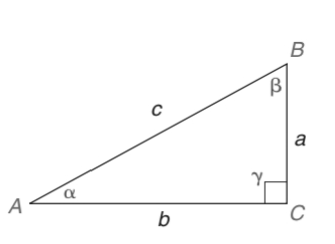Chapter 11.3, Problem 34E### Elementary Geometry for College St...

6th Edition
Daniel C. Alexander + 1 other
ISBN: 9781285195698

#### Solutions

Chapter
Section### Elementary Geometry for College St...

6th Edition
Daniel C. Alexander + 1 other
ISBN: 9781285195698
Textbook Problem
1 views

# In Exercises 33 to 36, we expand the list of trigonometric identities. As you may recall, an identity is a statement that is true for all permissible choices of the variable (see page 502.)a) For α ≠ 0 ∘ , prove the identity cot α = cos α sin α . b) Use your calculator to determine cot 57 ∘ by dividing cos 57 ∘ by sin 57 ∘ .To determine

(a)

To prove:

For α0, the identity cotα=cosαsinα.

Explanation

Given:

For α0, cotα=cosαsinα.

Definition used:

The trigonometric ratios:

Calculation:

Consider the angle α.

From the figure, the length of the opposite leg is a, the length of the adjacent leg is b and the hypotenuse is c.

sinα=ac

cosα=bc

cotα=1tanα=ba   ...(1)

Consider cosαsinα

To determine

(b)

To show:

The trigonometric ratio cot57 is equivalent to cos57sin57 using calculator.

### Still sussing out bartleby?

Check out a sample textbook solution.

See a sample solution

#### The Solution to Your Study Problems

Bartleby provides explanations to thousands of textbook problems written by our experts, many with advanced degrees!

Get Started

#### Expand each expression in Exercises 122. (x+1)(x3)

Finite Mathematics and Applied Calculus (MindTap Course List)

#### Sum of Square (SS), variance and standard deviation

Statistics for The Behavioral Sciences (MindTap Course List)

#### In Exercises 23-30, use logarithms to solve the equation for t. 2e0.2t4=6

Finite Mathematics for the Managerial, Life, and Social Sciences

#### π does not exist

Study Guide for Stewart's Multivariable Calculus, 8th